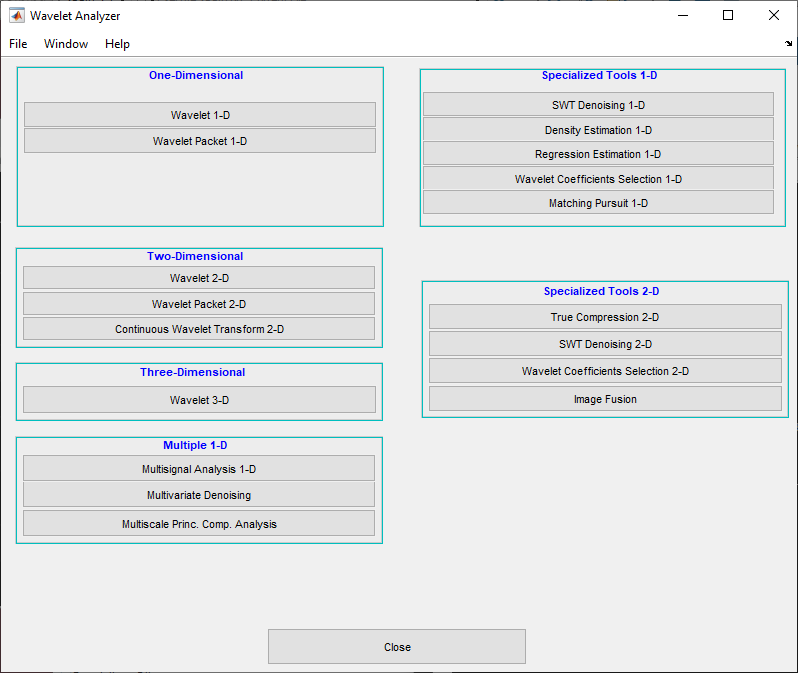## Image Fusion

For the example, switch the extension mode to symmetric padding, using the command:

```dwtmode('sym') ```

The toolbox requires only one function for image fusion: `wfusimg`. You'll find full information about this function in its reference page. For more details on fusion methods see the `wfusmat` function.

In this section, you will learn how to:

• Perform decompositions

• Merge images from their decompositions

• Restore images from their decompositions

• Save image after fusion

The principle of image fusion using wavelets is to merge the wavelet decompositions of the two original images using fusion methods applied to approximations coefficients and details coefficients (see [MisMOP03] and [Zee98] in References).

The two images must be of the same size and are supposed to be associated with indexed images on a common colormap (see `wextend` to resize images).

Two examples are examined: the first one merges two different images leading to a new image and the second restores an image from two fuzzy versions of an original image.

### Image Fusion Using the Command Line

#### Example 1: Fusion of Two Different Images

```load mask; X1 = X; load bust; X2 = X; ```
2. Merge the two images from wavelet decompositions at level 5 using `db2` by taking two different fusion methods: fusion by taking the mean for both approximations and details,

```XFUSmean = wfusimg(X1,X2,'db2',5,'mean','mean'); ```

and fusion by taking the maximum for approximations and the minimum for the details.

```XFUSmaxmin = wfusimg(X1,X2,'db2',5,'max','min'); ```
3. Plot original and synthesized images.

```colormap(map); subplot(221), image(X1), axis square, title('Mask') subplot(222), image(X2), axis square, title('Bust') subplot(223), image(XFUSmean), axis square, title('Synthesized image, mean-mean') subplot(224), image(XFUSmaxmin), axis square, title('Synthesized image, max-min') ```#### Example 2: Restoration by Fusion from Fuzzy Images

1. Load two fuzzy versions of an original image.

```load cathe_1; X1 = X; load cathe_2; X2 = X; ```
2. Merge the two images from wavelet decompositions at level 5 using `sym4` by taking the maximum of absolute value of the coefficients for both approximations and details.

```XFUS = wfusimg(X1,X2,'sym4',5,'max','max'); ```
3. Plot original and synthesized images.

```colormap(map); subplot(221), image(X1), axis square, title('Catherine 1') subplot(222), image(X2), axis square, title('Catherine 2') subplot(223), image(XFUS), axis square, title('Synthesized image') ```The synthesized image is a restored version of good quality of the common underlying original image.

### Image Fusion Using the Wavelet Analyzer App

The principle of image fusion using wavelets is to merge the wavelet decompositions of the two original images using fusion methods applied to approximations coefficients and details coefficients (see [MisMOP03] and [Zee98] in References).

The two images must be of the same size and are supposed to be associated with indexed images on a common colormap (see `wextend` to resize images).

Two examples are examined: the first one merges two different images leading to a new image and the second restores an image from two fuzzy versions of an original image.

1. Start the Wavelet Analyzer App.

From the MATLAB® prompt, type `waveletAnalyzer` to display the Wavelet Analyzer and then click the Image Fusion menu item to display the Image Fusion Tool.```load mask; X1 = X; load bust; X2 = X; ```
In the Image Fusion tool, select File > Load or Import Image 1 > Import from Workspace. When the Import from Workspace dialog box appears, select the `X1` variable, which loads the mask image.

Perform the same sequence choosing the `X2` variable to load the bust image.

3. Perform wavelet decompositions.

Using the Wavelet and Level menus located to the upper right, determine the wavelet family, the wavelet type, and the number of levels to be used for the analysis.

For this analysis, select the `db2` wavelet at level 5.

Click the Decompose button.

After a pause for computation, the tool displays the two analyses.4. Merge two images from their decompositions.

From Select Fusion Method frame, select the item `mean` for both Approx. and Details. Next, click the Apply button.The synthesized image and its decomposition (which is equal to the fusion of the two decompositions) appear. The new image produced by fusion clearly exhibits features from the two original ones.

Let us now examine another example illustrating restoration using image fusion.

5. Restore the image using image fusion.

From the File menu, load Image 1 by selecting the MAT-file `cathe_1.mat`, and Image 2 by selecting the MAT-file `cathe_2.mat`.

6. Using the Wavelet and Level menus, select the `sym4` wavelet at level 5. Click the Decompose button.

7. From Select Fusion Method frame, select the item `max` for both Approx. and Details. Next, click the Apply button.The synthesized image is a restored version of good quality of the common underlying original image.

### Saving the Synthesized Image

The Image Fusion Tool lets you save the synthesized image to disk. The toolbox creates a MAT-file in the current folder with a name you choose.

To save the synthesized image from the present selection, use the menu option File > Save Synthesized Image.

A dialog box appears that lets you specify a folder and filename for storing the image. After you save the image data to the file `rescathe.mat`, the synthesized image is given by `X` and the colormap by `map`.

## SupportGet trial now